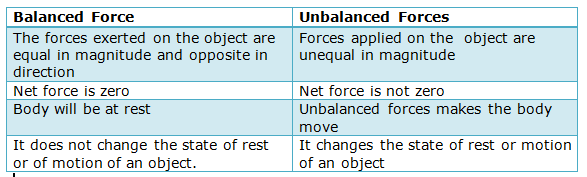Home » Physics » question on force

# Questions On Force with answers

On this page we are providing some frequently asked questions on the concept of force along with their answers. Please note that these questions on the concept of force are not numerical questions. These are some basic conceptual questions and students must be familiar with these concepts to solve numerical problems in this topic.

Notes on force and laws of motion (opens in new window).

Why do people sitting in bus tend to fall sideways when the bus takes a sharp u turn?

When the bus moves along a straight line our body also move with the same speed along the straight line but when the bus makes a sharp U-turn our body upper portion is still in the straight line motion but the lower portion of body move with the bus so we fall sideways when the bus takes sharp U-turn. This happens due to the inertia of direction.
Note: The tendency of a body to oppose any change in its direction of motion is known as the inertia of direction.

When balanced forces act on a stationary body, then the body is deformed True or False

False because balanced forces do not cause any change in the sate of the object.

What is inertia?

Inertia is a property of matter by which it continues in its existing state of rest or uniform motion in a straight line unless that state is changed by an external force.

Can a body have acceleration without a change in the magnitude of velocity? Explain with an example.

Yes, a body can have acceleration without any change in its velocity.
It is the case of Uniform Circular Motion in which centripetal acceleration works towards the center while the body has constant velocity or magnitude of velocity does not change.

State three laws of motion?

The first law of motion is stated as:
An object remains in a state of rest or of uniform motion in a straight line unless compelled to change that state by an applied force.
The second law of motion states that
the rate of change of momentum of an object is proportional to the applied unbalanced force in the direction of the force.
Newton’s third law of motion states that
Whenever a body exerts a force on another body, the second body exerts an equal and opposite force on the first body

Distinguish between balanced and unbalanced force? The resultant of balanced forces is non-zero True or false?False. The resultant balanced forces are always zero.
For more visit balanced and unbalanced forces

Why a person sitting in bus fall forward when moving bus suddenly stops?

This happens due to inertia of motion of upper part of his body. Here when moving bus suddenly stops, the lower part of the person’s body comes to rest with the bus while the upper part tends to continue its motion due to inertia.

What is impulse? Prove that Impulse is equal to change in momentum?

Product of Force and Time for which it is applied is called Impulse. SI unit of Impulse is $Ns$.
$Impulse=force \times time$
$Impulse=f \times t$
$\qquad=ma\times t$
$\qquad =m \frac{\Delta v}{t} \times t$
$\qquad =m \Delta v$
$\qquad =m(v-u)$
$\qquad =mv-mu$
This shows that impulse is equal to change in their linear momentum.

Define momentum and Prove that $F = ma$

Momentum can be defined as “mass in motion”. All objects have mass, so if an object is moving, then it has momentum i.e., it has its mass in motion.
The momentum, $p$ of an object is defined as the product of its mass, $m$ and velocity, $v$. That is,
momentum $p=mv$
For more in-depth discussion please visit proving F=ma?

Why dust fall on beating carpet with stick?

This happens because of law of inertia.
Dust particles present on surface of carpet are at rest but when we hit carpet with a stick the carpet cloth’s position changes but dust particles remain in their position due to inertia of rest and that’s why dust come out.

Question: Why is it difficult for a fireman to the hose, which ejects a large amount of water at a high velocity?

Question: The state of motion of an object is described by both its speed and the direction of motion. True or False?

Question: State and verify the law of conservation of momentum?

### Related Articles

Subscribe
Notify of

This site uses Akismet to reduce spam. Learn how your comment data is processed.